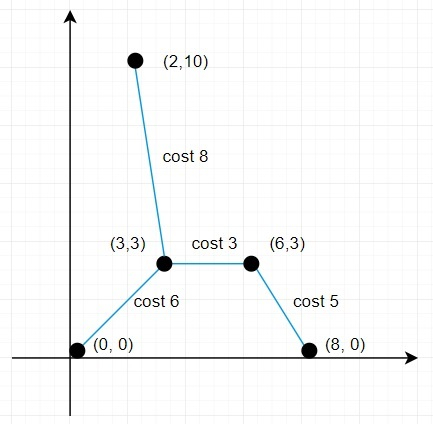# Program to find minimum cost to connect all points in Python

Suppose we have an array called points with some points in the form (x, y). Now the cost of connecting two points (xi, yi) and (xj, yj) is the Manhattan distance between them, the formula is |xi - xj| + |yi - yj|. We have to find the minimum cost to make all points connected.

So, if the input is like points = [(0,0),(3,3),(2,10),(6,3),(8,0)], then the output will be 22 becauseso here total distance is (6+5+3+8) = 22.

To solve this, we will follow these steps −

• points_set := a new set holding numbers from range 0 to size of points - 1
• heap := make a heap with pair (0, 0)
• visited_node := a new set
• total_distance := 0
• while heap is not empty and size of visited_node < size of points, do
• (distance, current_index) := delete element from heap
• if current_index is not present in visited_node, then
• insert current_index into visited_node
• delete current_index from points_set
• total_distance := total_distance + distance
• (x0, y0) := points[current_index]
• for each next_index in points_set, do
• (x1, y1) := points[next_index]
• insert (|x0 - x1| + |y0 - y1| , next_index) into heap

## Example

Let us see the following implementation to get better understanding −

import heapq
def solve(points):
points_set = set(range(len(points)))
heap = [(0, 0)]
visited_node = set()
total_distance = 0
while heap and len(visited_node) < len(points):
distance, current_index = heapq.heappop(heap)
if current_index not in visited_node:
total_distance += distance
x0, y0 = points[current_index]
for next_index in points_set:
x1, y1 = points[next_index]
heapq.heappush(heap, (abs(x0 - x1) + abs(y0 - y1), next_index))
print(solve(points))
[(0,0),(3,3),(2,10),(6,3),(8,0)]
22